The Australian Journal of Mathematical Analysis and Applications

 Home News Editors Volumes RGMIA Subscriptions Authors Contact

ISSN 1449-5910

Sorry death is imminent for file: ../public_html/searchroot/files/tex/v19n2/v19i2p4.tex
You searched for ravi
Total of 148 results found in site

13: Paper Source PDF document

Paper's Title:

On a Criteria for Strong Starlikeness

Author(s):

V. Ravichandran, M. Darus, and N. Seenivasagan

School Of Mathematical Sciences, Universiti Sains Malaysia,
11800 Usm Penang, Malaysia
vravi@cs.usm.my
URL: http://cs.usm.my/~vravi

School of Mathematical Sciences, Faculty of Sciences and Technology,
Ukm, Bangi 43600, Malaysia
maslina@pkrisc.cc.ukm.my

Sindhi College, 123, P. H. Road, Numbal,
Chennai 600 077 India
vasagan2000@yahoo.co.in

Abstract:

In this paper, we are concerned with finding sufficient condition for certain normalized analytic function f(z) defined on the open unit disk in the complex plane to be strongly starlike of order α. Also we have obtained similar results for certain functions defined by Ruscheweyh derivatives and Sălăgean derivatives. Further extension of these results are given for certain p-valent analytic functions defined through a linear operator.

7: Paper Source PDF document

Paper's Title:

Fekete-Szegö Inequality for Certain Class of Analytic Functions

Author(s):

V. Ravichandran, Maslina Darus, M. Hussain Khan, and  K. G. Subramanian

School of Mathematical Sciences, Universiti Sains Malaysia,
11800 Usm, Penang, Malaysia

School of Mathematical Sciences, Faculty of Sciences and Technology,
Ukm, Banki 43600, Malaysia

Department of Mathematics, Islamiah College,
V

Department of Mathematics, Madras Christian College, Tambaram,
Chennai- 600 059, India

Abstract:

In this present investigation, the authors obtain Fekete-Szegö inequality for a certain class of analytic functions f(z) for which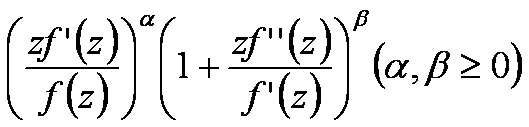lies in a region starlike with respect to 1 and symmetric with respect to the real axis. Also certain application of our main result for a class of functions defined by Hadamard product (convolution) is given. As a special case of our result we obtain Fekete-Szegö inequality for a class of functions defined through fractional derivatives. Also we obtain Fekete-Szegö inequality for the inverse functions.

7: Paper Source PDF document

Paper's Title:

Equivalence of the Nonsmooth Nonlinear Complementarity Problems to Unconstrained Minimization

Author(s):

M. A. Tawhid and J. L. Goffin

Department of Mathematics and Statistics, School of Advanced Technologies and Mathematics,
Thompson Rivers University,
900 McGill Road, PO Box 3010, Kamloops, BC V2C 5N3
Alexandria University and Egypt Japan University of Science and Technology,
Alexandria-Egypt
mtawhid@tru.ca

Faculty of Management, McGill University,
1001 Sherbrooke Street West, Montreal, Quebec, H3A 1G5
Jean-Louis.Goffin@McGill.ca

Abstract:

This paper deals with nonsmooth nonlinear complementarity problem, where the underlying functions are nonsmooth which admit the H-differentiability but not necessarily locally Lipschitzian or directionally differentiable. We consider a reformulation of the nonlinear complementarity problem as an unconstrained minimization problem. We describe H-differentials of the associated penalized Fischer-Burmeister and Kanzow and Kleinmichel merit functions. We show how, under appropriate P0, semimonotone (E0), P, positive definite, and strictly semimonotone (E) -conditions on an H-differential of f, finding local/global minimum of a merit function (or a `stationary point' of a merit function) leads to a solution of the given nonlinear complementarity problem. Our results not only give new results but also unify/extend various similar results proved for C1.

6: Paper Source PDF document

Paper's Title:

A Coefficient Inequality For Certain Subclasses of Analytic Functions Related to Complex Order

Author(s):

B. Srutha Keerthi, B. Adolf Stephen and S. Sivasubramanian

Department Of Applied Mathematics, Sri Venkateswara College Of Engineering, Anna University,
Sriperumbudur, Chennai - 602 105,
India.
laya@svce.ac.in

Department of Mathematics, Madras Christian College, Chennai - 600059,
India

Department of Mathematics, College of Engineering, Anna University,
India.
sivasaisastha@rediffmail.com

Abstract:

In this present investigation, the authors obtain coefficient inequality for certain normalized analytic functions of complex order f(z) defined on the open unit disk for which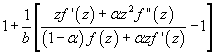(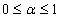and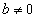be a complex number) lies in a region starlike with respect to 1 and is symmetric with respect to the real axis. Also certain applications of the main result for a class of functions of complex order defined by convolution are given. As a special case of this result, coefficient inequality for a class of functions defined through fractional derivatives is obtained. The motivation of this paper is to give a generalization of the coefficient inequalities of the subclasses of starlike and convex functions of complex order.

6: Paper Source PDF document

Paper's Title:

A Note on the Ulam stability of Reciprocal Difference and Adjoint Functional Equations

Author(s):

K. Ravi, J. M. Rassias, M. E. Gordji, and B. V. Senthil Kumar

Department of Mathematics,
Sacred Heart College, Tirupattur - 635601,
India
shckavi@yahoo.co.in

Pedagogical Department E. E.,
Section of Mathematics and Informatics,
National and Capodistrian University of Athens,
Athens, Attikis 15342,
GREECE
jrassias@primedu.uoa.gr

Department of Mathematics, Semnan University,
P.O. Box 35195-363, Semnan,
Iran

Department of Mathematics,
C.Abdul Hakeem College of Engineering and
Technology, Melvisharam - 632 509,
India
bvssree@yahoo.co.in

Abstract:

This note is an erratum to previous work published as Volume 8, Issue 1, Paper 13, 2011 of The Australian Journal of Mathematical Analysis and Applications.

6: Paper Source PDF document

Paper's Title:

A Low Order Least-Squares Nonconforming Finite Element Method for Steady Magnetohydrodynamic Equations

Author(s):

Z. Yu, D. Shi and H. Zhu

College of Science,
Zhongyuan University of Technology,
Zhengzhou 450007,
China.
E-mail: 5772@zut.edu.cn

School of Mathematics and Statistics,
Zhengzhou University,
Zhengzhou 450001,
China.
E-mail: shi_dy@126.com

Mathematics Department,
University of Southern Mississippi,
Hattiesburg MS, 39406,
U.S.A
E-mail: huiqing.zhu@usm.edu

Abstract:

A low order least-squares nonconforming finite element (NFE) method is proposed for magnetohydrodynamic equations with EQ1rot element and zero-order Raviart-Thomas element. Based on the above element's typical interpolations properties, the existence and uniqueness of the approximate solutions are proved and the optimal order error estimates for the corresponding variables are derived.

5: Paper Source PDF document

Paper's Title:

Differential Sandwich Theorems for Some Subclasses of Analytic Functions

Author(s):

T. N. Shanmugam, V. Ravichandran and S. Sivasubramanian

Department of Mathematics, College of Engineering,
Anna university, Chennai 600 025,
India
shan@annauniv.edu
URL: http://www.annauniv.edu/shan

School of Mathematical Sciences,
Universiti Sains Malaysia,
11800 USM Penang,
Malaysia
vravi@cs.usm.my
URL: http://cs.usm.my/~vravi

Department of Mathematics, Easwari Engineering college,
Ramapuram, Chennai 600 089,
India
sivasaisastha@rediffmail.com

Abstract:

Let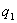and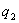be univalent in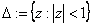with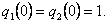We give some applications of first order differential subordination and superordination to obtain sufficient conditions for normalized analytic function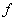with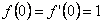to satisfy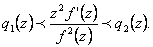5: Paper Source PDF document

Paper's Title:

On the Numerical Solution for Deconvolution Problems with Noise

Author(s):

N. H. Sweilam

Cairo University, Faculty of Science, Mathematics Department,
Giza, Egypt.
n-sweilam@yahoo.com

Abstract:

In this paper three different stable methods for solving numerically deconvolution problems with noise are studied. The methods examined are the variational regularization method, the dynamical systems method, and the iterative regularized processes. Gravity surveying problem with noise is studied as a model problem. The results obtained by these methods are compared to the exact solution for the model problem. It is found that these three methods are highly stable methods and always converge to the solution even for large size models. The relative higher accuracy is obtained by using the iterative regularized processes.

5: Paper Source PDF document

Paper's Title:

On Sandwich Theorems for Certain Subclass of Analytic Functions Involving Dziok-Srivastava Operator

Author(s):

T. N. Shanmugam, M. P. Jeyarama and A. Singaravelu

Department of Mathematics
College of Engineering, Anna University
Chennai - 600 025,
India
drtns2001@yahoo.com

Department of Mathematics
Easwari Engineering College
Ramapuram, Chennai - 600089
jeyaraman-mp@yahoo.co.i

Department of Mathematics
Valliammai Engineering College
Chennai - 603203
asing-59@yahoo.com

Abstract:

The purpose of this present paper is to derive some subordination and superordination results for certain normalized analytic functions in the open unit disk, acted upon by Dziok-Srivastava operator. Relevant connections of the results, which are presented in this paper, with various known results are also considered.

5: Paper Source PDF document

Paper's Title:

Ulam Stability of Reciprocal Difference and Adjoint Functional Equations

Author(s):

K. Ravi, J. M. Rassias and B. V. Senthil Kumar

Department of Mathematics,
Sacred Heart College, Tirupattur - 635601,
India

Pedagogical Department E. E.,
Section of Mathematics and Informatics,
National and Capodistrian University of Athens,
Athens, Attikis 15342,
GREECE

Department of Mathematics,
C.Abdul Hakeem College of Engineering and
Technology, Melvisharam - 632 509, India

shckavi@yahoo.co.in
jrassias@primedu.uoa.gr
bvssree@yahoo.co.in

Abstract:

In this paper, the reciprocal difference functional equation (or RDF equation) and the reciprocal adjoint functional equation (or RAF equation) are introduced. Then the pertinent Ulam stability problem for these functional equations is solved, together with the extended Ulam (or Rassias) stability problem and the generalized Ulam (or Ulam-Gavruta-Rassias) stability problem for the same equations.

5: Paper Source PDF document

Paper's Title:

Coefficient Bounds for Sakaguchi Kind of Functions Associated with Sine Function

Author(s):

Serap Bulut, H. Priya and B. Srutha Keerth

Kocaeli University,
Faculty of Aviation and Space Sciences,
Arslanbey Campus, 41285 Kartepe-Kocaeli,
Turkey.
E-mail: serap.bulut@kocaeli.edu.tr

Department of Mathematics,
VIT Chennai Campus, Chennai - 600 048,
India.
E-mail: priyaharikrishnan18@gmail.com, priya.h2020@vitstudent.ac.in

Department of Mathematics,
VIT Chennai Campus, Chennai - 600 048,
India.
E-mail: keerthivitmaths@gmail.com, sruthakeerthi.b@vit.ac.in

Abstract:

In this paper, we introduce a new general subclass of analytic functions with respect to symmetric points in the domain of sine function. We obtain sharp coefficient bounds and upper bounds for the Fekete-Szegö functional. Also we get sharp bounds for the logarithmic coefficients of functions belonging to this new class.

4: Paper Source PDF document

Paper's Title:

Classes of Meromorphic p-valent Parabolic Starlike Functions with Positive Coefficients

Author(s):

S. Sivaprasad Kumar, V. Ravichandran, and G. Murugusundaramoorthy

Department of Applied Mathematics
Delhi College of Engineering,
Delhi 110042, India
sivpk71@yahoo.com

School of Mathematical Sciences
Universiti Sains Malaysia
11800 USM Penang
Malaysia
vravi@cs.usm.my
URL: http://cs.usm.my/~vravi

Department of Mathematics
Vellore Institute of Technology (Deemed University)
Vellore 632 014, India
gmsmoorthy@yahoo.com

Abstract:

In the present paper, we consider two general subclasses of meromorphic p-valent starlike functions with positive coefficients and obtain a necessary and sufficient condition for functions to be in these classes. Also we obtain certain other related results as a consequences of our main results.

4: Paper Source PDF document

Paper's Title:

Certain Coefficient Estimates for Bi-univalent Sakaguchi Type Functions

Author(s):

B. Srutha Keerthi, S. Chinthamani

Department of Applied Mathematics,
Sri Venkateswara College of Engineering,
Sriperumbudur, Chennai - 602105,
India

Abstract:

Estimates on the initial coefficients are obtained for normalized analytic functions f in the open unit disk with f and its inverse g = f-1 satisfying the conditions that zf'(z) / f(z) and zg'(z) / g(z) are both subordinate to a starlike univalent function whose range is symmetric with respect to the real axis. Several related classes of functions are also considered, and connections to earlier known results are made.

4: Paper Source PDF document

Paper's Title:

Hankel Functional Connected to Lemniscate of Bernoulli

Author(s):

K. Ramanuja Rao, Rajnesh Lal and Kaushal Singh

Fiji National University,
Department of Mathematics & Statistics,
P.O. Box 5529, Lautoka,
Fiji.
E-mail: ramanuja.kotti@fnu.ac.fj
rajnesh.lal@fnu.ac.fj
kaushal.singh@fnu.ac.fj

Abstract:

The aim of present paper is to derive a higher bound (HB) of 3rd order Hankel determinant for a collection of holomorphic mappings connected with exactly to the right side of the lemniscate of Bernoulli, whose polar coordinates form is r2 = 2cos2(2θ). The method carried in this paper is more refined than the method adopted by the authors (see ), who worked on this problem earlier.

3: Paper Source PDF document

Paper's Title:

On Sufficient Conditions for Strong Starlikeness

Author(s):

V. Ravichandran, M. H. Khan, M. Darus, And K. G. Subramanian

School of Mathematical Sciences, Universiti Sains Malaysia, 11800 Usm Penang, Malaysia
Url
: h
ttp://cs.usm.my/~vravi/index.html

Department of Mathematics, Islamiah College, Vaniambadi 635 751, India
khanhussaff@yahoo.co.in

School of Mathematical Sciences, Faculty of Science and Technology, UKM, Bangi 43600,
M
alaysia
maslina@pkrisc.cc.ukm.my
Url
:

Department of Mathematics, Madras Christian College, Tambaram, Chennai 600 059, India

Abstract:

In the present investigation, we obtain some sufficient conditions for a normalized analytic function f(z) defined on the unit disk to satisfy the condition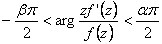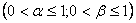3: Paper Source PDF document

Paper's Title:

On the Fekete-Szegő Inequality for Some Subclasses of Analytic Functions

Author(s):

T.N. Shanmugam and A. Singaravelu

Department of Mathematics,
College of Engineering,
Anna University, Chennai-600 025,
shan@annauniv.edu

Department of Mathematics,
Valliammai Engineering College,
Chennai-603 203,
sivasaisastha@rediffmail.com

Abstract:

In this present investigation, the authors obtainFekete-Szegő's inequality for certain normalized analytic functions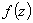defined on the open unit disk for which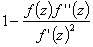lie in a region starlike with respect to 1 and symmetric with respect to the real axis. Also certain applications of the main result for a class of functions defined by convolution are given. As a special case of this result, Fekete-Szegő's inequality for a class of functions defined through fractional derivatives is also obtained.

3: Paper Source PDF document

Paper's Title:

Some Inequalities for a Certain Class of Multivalent Functions Using Multiplier Transformation

Author(s):

Department Of Applied Mathematics
Sri Venkateswara College Of Engineering
Sriperumbudur, Chennai - 602105,
India.
suchithravenkat@yahoo.co.in

Department Of Mathematics,
Chennai - 600059,
India.

Department Of Applied Mathematics
Sri Venkateswara College Of Engineering
Sriperumbudur, Chennai - 602105,
India.
ganga@svce.ac.in

Department Of Mathematics,
Easwari Engineering College
Ramapuram, Chennai - 600089,
India.
ganga@svce.ac.in

Abstract:

The object of the present paper is to derive several inequalities associated with differential subordinations between analytic functions and a linear operator defined for a certain family of p-valent functions, which is introduced here by means of a family of extended multiplier transformations. Some special cases and consequences of the main results are also considered.

3: Paper Source PDF document

Paper's Title:

Fekete-Szegö Problem for Univalent Functions with Respect to k-Symmetric Points

Author(s):

K. Al-Shaqsi and M. Darus

School of Mathematical Sciences, Faculty of Science and Technology,
University Kebangsaan Malaysia,
Bangi 43600 Selangor D. Ehsan,
Malaysia
ommath@hotmail.com
maslina@ukm.my

Abstract:

In the present investigation, sharp upper bounds of |a3- μa22| for functions f(z) = z + a2z2 + a2z3 + ... belonging to certain subclasses of starlike and convex functions with respect to k-symmetric points are obtained. Also certain applications of the main results for subclasses of functions defined by convolution with a normalized analytic function are given. In particular, Fekete- Szegö inequalities for certain classes of functions defined through fractional derivatives are obtained.

3: Paper Source PDF document

Paper's Title:

Hyperbolic Barycentric Coordinates

Author(s):

Abraham A. Ungar

Department of Mathematics, North Dakota State University,
Fargo, ND 58105,
USA
Abraham.Ungar@ndsu.edu
URL
: http://math.ndsu.nodak.edu/faculty/ungar/

Abstract:

A powerful and novel way to study Einstein's special theory of relativity and its underlying geometry, the hyperbolic geometry of Bolyai and Lobachevsky, by analogies with classical mechanics and its underlying Euclidean geometry is demonstrated. The demonstration sets the stage for the extension of the notion of barycentric coordinates in Euclidean geometry, first conceived by Möbius in 1827, into hyperbolic geometry. As an example for the application of hyperbolic barycentric coordinates, the hyperbolic midpoint of any hyperbolic segment, and the centroid and orthocenter of any hyperbolic triangle are determined.

3: Paper Source PDF document

Paper's Title:

Subordination Results Associated with Hadamard Product

Author(s):

S. Sivasubramanian, C. Ramachandran and B. A. Frasin

Department of Mathematics,
University College of Engineering,
Anna University,
Saram-604 307,
India

sivasaisastha@rediffmail.com

Department of Mathematics,
University College of Engineering,
Anna University,
Villupuram,
India

crjsp2004@yahoo.com

Department of Mathematics,
Al al-Bayt University,
P.O. Box: 130095 Mafraq,
Jordan

Abstract:

In the present investigation, we consider an unified class of functions of complex order using Hadamard's convolution. We obtain a necessary and sufficient condition for functions to be in these classes.

3: Paper Source PDF document

Paper's Title:

Residual-Based A Posteriori Error Estimates For A Conforming Mixed Finite Element Discretization of the Monge-Ampere Equation

Author(s):

J. Adetola, K. W. Houedanou and B. Ahounou

Institut de Mathematiques et de Sciences Physiques (IMSP),
Universite d'Abomey-Calavi

Departement de Mathematiques,
Faculte des Sciences et Techniques (FAST),
Universite d'Abomey-Calavi
E-mail: khouedanou@yahoo.fr

Departement de Mathematiques,
Faculte des Sciences et Techniques (FAST),
Universite d'Abomey-Calavi
E-mail: bahounou@yahoo.fr

Abstract:

In this paper we develop a new a posteriori error analysis for the Monge-Ampere equation approximated by conforming finite element method on isotropic meshes in R2. The approach utilizes a slight variant of the mixed discretization proposed by Gerard Awanou and Hengguang Li in . The a posteriori error estimate is based on a suitable evaluation on the residual of the finite element solution. It is proven that the a posteriori error estimate provided in this paper is both reliable and efficient.

3: Paper Source PDF document

Paper's Title:

Numerical Study of a Mathematical Model of a Free-Surface Potential Flow

Author(s):

H. Serguine, F. Guechi and A. Gasmi

Department Of Mathematics, Faculty of Science,
Ferhat Abbas Universty, 19000, Setif,
Algeria.
E-mail: houria.serguine@univ-msila.dz

Department Of Mathematics, Faculty of Science,
Ferhat Abbas Universty, 19000, Setif,
Algeria.
E-mail:  fairouz.chegaar@univ-setif.dz

Laboratory of Pure and Applied Mathematics, Faculty of Mathematics and Computer Science,
Mohamed Boudiaf Universty, 28000, M'sila,
Algeria.

Abstract:

In this work, the problem of a potential and two-dimensional flow with a free surface of an incompressible, irrotational and inviscid fluid of a jet in front an inclined wall is considered, where γ is the inclination angle with the horizontal. The shape of the free surface is presented by curves which are found numerically by the series truncation method. This technique is based on the conformal transformations, resulting with the surface tension effect T with the boundary conditions on the free surfaces given by Bernoulli's equation. The found results are dependant on parameters which are: the Weber's number α and the angle γ. For each Weber's number value, only one solution is specified and some shapes of free surfaces of the jet are illustrated.

2: Paper Source PDF document

Paper's Title:

Generalized Hypergeometric Functions Defined on the Class of Univalent Functions

Author(s):

N. Marikkannan, A. Gangadharan and C. Ganesamoorthy

Department of Applied Mathematics,
Sri Venkateswara College of Engineering,
Sriperumbudur 602105,
India.
mari@svce.ac.in

Department of Applied mathematics,
Sri Venkateswara College of Engineering,
Sriperumbudur 602105,
India.
ganga@svce.ac.in

Department of Mathematics,
Alagappa university,
Karaikudi,
India.
ganesamoorthyc@yahoo.com

Abstract:

Let A denotes the class of all analytic functions f(z), normalized by the condition f'(0)-1=f(0)=0 defined on the open unit disk Δ and S be the subclass of A containing univalent functions of A. In this paper, we find the sufficient conditions for hypergeometric functions defined on S to be in certain subclasses of A, like k-UCV, k-ST

2: Paper Source PDF document

Paper's Title:

An Approximation of Jordan Decomposable Functions for a Lipschitz Function

Author(s):

Ibraheem Alolyan

Mathematics Department,
College of Science, King Saud University
Saudi Arabia
ialolyan05@yahoo.com

Abstract:

The well known Jordan decomposition theorem gives the useful characterization that any function of bounded variation can be written as the difference of two increasing functions. Functions which can be expressed in this way can be used to formulate an exclusion test for the recent Cellular Exclusion Algorithms for numerically computing all zero points or the global minima of functions in a given cellular domain [2,8,9]. In this paper we give an algorithm to approximate such increasing functions when only the values of the function of bounded variation can be computed. For this purpose, we are led to introduce the idea of ε-increasing functions. It is shown that for any Lipschitz continuous function, we can find two ε-increasing functions such that the Lipschitz function can be written as the difference of these functions.

2: Paper Source PDF document

Paper's Title:

Oscillation and Boundedness of Solutions to First and Second Order Forced Dynamic Equations with Mixed Nonlinearities

Author(s):

Ravi P. Agarwal and Martin Bohner

Department of Mathematical Sciences, Florida Institute of Technology
Melbourne, FL 32901,
U.S.A.
bohner@mst.edu
URL:http://web.mst.edu/~bohner

Department of Economics and Finance, Missouri University of Science and Technology
Rolla, MO 65401,
U.S.A.
agarwal@fit.edu

Abstract:

Some oscillation and boundedness criteria for solutions to certain first and second order forced dynamic equations with mixed nonlinearities are established. The main tool in the proofs is an inequality due to Hardy, Littlewood and Pólya. The obtained results can be applied to differential equations, difference equations and q-difference equations. The results are illustrated with numerous examples.

2: Paper Source PDF document

Paper's Title:

Sufficient Conditions for Certain Types of Functions to be Parabolic Starlike

Author(s):

Department of Mathematics,
Easwari Engineering College,
Ramapuram, Chennai - 89,
India.

Research Scholar,
Anna University,
Chennai

E-mail: ganga.megalai@gmail.com

E-mail: chinvicky@rediffmail.com

Abstract:

In this paper sufficient conditions are determined for functions of the form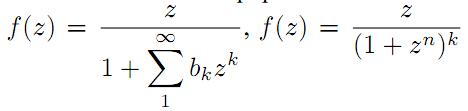and certain other types of functions to be parabolic starlike.

2: Paper Source PDF document

Paper's Title:

A New Relaxed Complex-valued b-metric Type and Fixed Point Results

Author(s):

P. Singh, V. Singh and T. C. M. Jele

Department of Mathematics, University of KwaZulu-Natal,
Private Bag X54001, Durban,
South Africa.
E-mail: singhp@ukzn.ac.za
singhv@ukzn.ac.za
thokozani.jele@nwu.ac.za

Abstract:

In this paper, we study the existence and uniqueness of fixed point in complex valued b-metric spaces and introduce a new relaxed α, β Complex-valued b-metric type by relaxing the triangle inequality and determine whether the fixed point theorems are applicable in these spaces.

2: Paper Source PDF document

Paper's Title:

Oscillatory Behavior of Second-Order Non-Canonical Retarded Difference Equations

Author(s):

G.E. Chatzarakis1, N. Indrajith2, E. Thandapani3 and K.S. Vidhyaa4

1Department of Electrical and Electronic Engineering Educators,
School of Pedagogical and Technological Education,
Marousi 15122, Athens,
Greece.
E-mail:  gea.xatz@aspete.gr, geaxatz@otenet.gr

2Department of Mathematics,
Presidency College, Chennai - 600 005,
India.
E-mail: indrajithna@gmail.com

3Ramanujan Institute for Advanced Study in Mathematics,
Chennai - 600 005,
India.
E-mail: ethandapani@yahoo.co.in

4Department of Mathematics,
SRM Easwari Engineering College,
Chennai-600089,
India.
E-mail: vidyacertain@gmail.com

Abstract:

Using monotonic properties of nonoscillatory solutions, we obtain new oscillatory criteria for the second-order non-canonical difference equation with retarded argument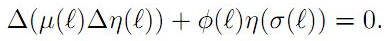Our oscillation results improve and extend the earlier ones. Examples illustrating the results are provided.

2: Paper Source PDF document

Paper's Title:

A Generalization of a Partial b-metric and Fixed Point Theorems

Author(s):

Pravin Singh and Virath Singh

Department of Mathematics, University of KwaZulu-Natal,
Private Bag X54001, Durban,
South Africa.
E-mail: singhp@ukzn.ac.za
singhv@ukzn.ac.za

Abstract:

The purpose of this paper is to introduce the concept of a Partial α, β b-metric as a generalization of a partial b-metric and prove theorems for some contractive type mapping.

2: Paper Source PDF document

Paper's Title:

Fekete-Szegö Inequality for Sakaguchi Type of functions in Petal Shaped Domain

Author(s):

E. K. Nithiyanandham and B. Srutha Keerthi

Division of Mathematics, School of Advanced Sciences,
Vellore Institute of Technology Chennai Campus,
Chennai - 600 048,
India.
E-mail: nithiyankrish@gmail.com

Division of Mathematics, School of Advanced Sciences,
Vellore Institute of Technology Chennai Campus,
Chennai - 600 048,
India.
E-mail: keerthivitmaths@gmail.com

Abstract:

In this paper, we estimate coefficient bounds,|a_2|,|a_3| and |a_4|, Fekete-Szegö inequality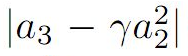and Toeplitz determinant T2(2) and T3(1) for functions belonging to the following class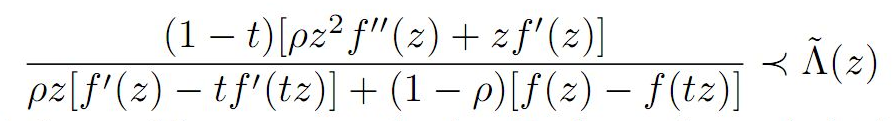the function being holomorphic, we expand using Taylor series and obtain several corollaries and consequences for the main result.

1: Paper Source PDF document

Paper's Title:

An Easy and Efficient Way for Solving A class of Singular Two Point Boundary Value Problems

Author(s):

Muhammed I. Syam, Muhammed N. Anwar and Basem S. Attili

Mathematical Sciences Department
United Arab Emirates University, P. O. Box 17551
Al-Ain, United Arab Emirates
b.attili@uaeu.ac.ae

Abstract:

We will consider an efficient and easy way for solving a certain class of singular two point boundary value problems. We will employ the least squares method which proved to be efficient for this type of problems. Enough examples that were considered by others will be solved with comparison with the results presented there.

1: Paper Source PDF document

Paper's Title:

A Reverse of the Triangle Inequality in Inner Product Spaces and Applications for Polynomials

Author(s):

I. Brnetić, S. S. Dragomir, R. Hoxha and J. Pečarić

Department of Applied Mathematics, Faculty of Electrical Engineering and Computing,
University of Zagreb, Unska 3, 10 000 Zagreb,
Croatia
andrea@zpm.fer.hr

School of Computer Science & Mathematics, Victoria University
Po Box 14428, Melbourne Vic 8001
Australia
sever.dragomir@vu.edu.au
URL:http://rgmia.vu.edu.au/dragomir

Faculty of Applied Technical Sciences, University of Prishtina,
Mother Theresa 5, 38 000 Prishtina
Kosova
razimhoxha@yahoo.com

Faculty of Textile Technology, University of Zagreb,
Pierottijeva 6, 10000 Zagreb,
Croatia
pecaric@hazu.hr

Abstract:

A reverse of the triangle inequality in inner product spaces related to the celebrated Diaz-Metcalf inequality with applications for complex polynomials is given.

1: Paper Source PDF document

Paper's Title:

A Subclass of Meromorphically Multivalent Functions with Applications to Generalized Hypergeometric Functions

Author(s):

M. K. Aouf

Mathematics Department, Faculty of Science,
Mansoura University 35516,
Egypt
mkaouf127@yahoo.com

Abstract:

In this paper a new subclass of meromorphically multivalent functions, which is defined by means of a Hadamard product (or convolution) involving some suitably normalized meromorphically p-valent functions. The main object of the present paper is to investigate the various important properties and characteristics of this subclass of meromorphically multivalent functions. We also derive many interesting results for the Hadamard products of functions belonging to this subclass. Also we consider several applications of our main results to generalized hypergeomtric functions.

1: Paper Source PDF document

Paper's Title:

Inclusion and Neighborhood Properties for Certain Subclasses of Analytic Functions Associated with Convolution Structure

Author(s):

M. K. Aouf

Mathematics Department,
Faculty of Science,
Mansoura University 35516,
Egypt.
mkaouf127@yahoo.com

Abstract:

In this paper we introduce and investigate two new subclasses of multivalently analytic functions of complex order defined by using the familiar convolution structure of analytic functions. In this paper we obtain the coefficient estimates and the consequent inclusion relationships involving the neighborhoods of the p-valently analytic functions.

1: Paper Source PDF document

Paper's Title:

Uniform Convergence of Schwarz Method for Noncoercive Variational Inequalities Simple Proof

Author(s):

Department of mathematics, LANOS Laboratory,
Faculty of the Sciences, University Badji Mokhtar,
P.O 23000 Annaba,
Algeria.

haiourm@yahoo.fr,

Abstract:

In this paper we study noncoercive variational inequalities, using the Schwarz method. The main idea of this method consists in decomposing the domain in two subdomains. We give a simple proof for the main result concerning L error estimates, using the Zhou geometrical convergence and the L approximation given for finite element methods by Courty-Dumont.

1: Paper Source PDF document

Paper's Title:

Certain Compact Generalizations of Well-Known Polynomial Inequalities

Author(s):

N. A. Rather and Suhail Gulzar

Department of Mathematics,
University of Kashmir, Hazratbal Srinagar-190006,
India.

Abstract:

In this paper, certain sharp compact generalizations of well-known Bernstien-type inequalities for polynomials, from which a variety of interesting results follow as special cases, are obtained.

1: Paper Source PDF document

Paper's Title:

Schwarz Method for Variational Inequalities Related to Ergodic Control Problems

Author(s):

Department of Mathematics, Badji Mokhtar University, Annaba 23000,
P.O.Box. 12, Annaba 23000, Algeria

signor_2000@yahoo.fr

Mcherihalima@yahoo.fr

Abstract:

In this paper, we study variational inequalities related to ergodic control problems studied by M. Boulbrachčne and H. Sissaoui , where the "discount factor" (i.e., the zero order term) is set to 0, we use an overlapping Schwarz method on nomatching grid which consists in decomposing the domain in two subdomains. For α ∈ ]0.1[ we provide the discretization on each subdomain converges in L -norm.

1: Paper Source PDF document

Paper's Title:

L∞- Error Estimate of Schwarz Algorithm for Elliptic Quasi-Variational Inequalities Related to Impulse Control Problem

Author(s):

Lab. LANOS, Department of Mathematics,
P.O.Box 12, Annaba 23000,
Algeria.

Lab. LAIG, Department of Mathematics,
University May 8th 1945,
P.O.Box 401, Guelma 24000,
Algeria.

allmehri@yahoo.fr

Abstract:

In this work, we study Schwarz method for a class of elliptic quasi-variational inequalities. The principal result of this investigation is to prove the error estimate in ∞-norm for two domains with overlapping nonmatching grids, using the geometrical convergence, and the uniform convergence of Cortey Dumont.

1: Paper Source PDF document

Paper's Title:

Ostrowski Type Inequalities for Lebesgue Integral: a Survey of Recent Results

Author(s):

Sever S. Dragomir1,2

1Mathematics, School of Engineering & Science
Victoria University, PO Box 14428
Melbourne City, MC 8001,
Australia
E-mail: sever.dragomir@vu.edu.au

2DST-NRF Centre of Excellence in the Mathematical and Statistical Sciences,
School of Computer Science & Applied Mathematics,
University of the Witwatersrand,
Private Bag 3, Johannesburg 2050,
South Africa
URL: http://rgmia.org/dragomir

Abstract:

The main aim of this survey is to present recent results concerning Ostrowski type inequalities for the Lebesgue integral of various classes of complex and real-valued functions. The survey is intended for use by both researchers in various fields of Classical and Modern Analysis and Mathematical Inequalities and their Applications, domains which have grown exponentially in the last decade, as well as by postgraduate students and scientists applying inequalities in their specific areas.

1: Paper Source PDF document

Paper's Title:

Introducing the Dorfmanian: A Powerful Tool for the Calculus Of Variations

Author(s):

Olivier de La Grandville

Department of Management Science and Engineering,
Stanford University,
475 Via Ortega, Stanford, CA 94305,
U. S. A.

E-mail: odelagrandville@gmail.com

Abstract:

We show how a modified Hamiltonian proposed by Robert Dorfman  to give intuitive sense to the Pontryagin maximum principle can be extended to easily obtain all high-order equations of the calculus of variations. This new concept is particularly efficient to determine the differential equations leading to the extremals of functionals defined by n-uple integrals, while a traditional approach would require -- in some cases repeatedly -- an extension of Green's theorem to n-space.
Our paper is dedicated to the memory of Robert Dorfman (1916 - 2002).

1: Paper Source PDF document

Paper's Title:

On Closed Range C*-modular Operators

Author(s):

Department of Mathematics,
Faculty of Mathematics and Statistics Sciences,
University of Birjand, Birjand,
Iran.

Abstract:

In this paper, for the class of the modular operators on Hilbert C*-modules, we give the conditions to closedness of their ranges. Also, the equivalence conditions for the closedness of the range of the modular projections on Hilbert C*-modules are discussed. Moreover, the mixed reverse order law for the Moore-Penrose invertible modular operators are given.

1: Paper Source PDF document

Paper's Title:

Action of Differential Operators On Chirpsconstruct On L

Author(s):

Taoufik El Bouayachi and Naji Yebari

Laboratoire de Mathematiques et applications,
Faculty of sciences and techniques, Tangier,
Morocco.
E-mail: figo407@gmail.com, yebarinaji@gmail.com

Abstract:

We will study in this work the action of differential operators on L chirps and we will give a new definition of logarithmic chirp. Finally we will study the action of singular integral operators on chirps by wavelet characterization and Kernel method.

1: Paper Source PDF document

Paper's Title:

On a subset of Bazilevic functions

Author(s):

Marjono and D. K. Thomas

Department of Mathematics,
Faculty of Mathematics and Natural Sciences,
Brawajaya University,
Malang, Jawa Timur 65145,
Indonesia.
E-mail: marjono@ub.ac.id

Department of Mathematics,
Swansea University, Singleton Park,
Swansea, SA2 8PP,
United Kingdom.
E-mail: d.k.thomas@swansea.ac.uk

Abstract:

Let S denote the class of analytic and univalent functions in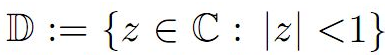of the form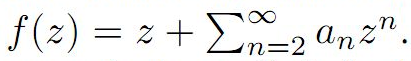For α≥0, the subclass B1α of S of Bazilevic functions has been extensively studied. In this paper we determine various properties of a subclass of B1α, for α≥0 which extends early results of a class of starlike functions studied by Ram Singh.

1: Paper Source PDF document

Paper's Title:

Some fixed point results in partial S-metric spaces

Author(s):

M. M. Rezaee, S. Sedghi, A. Mukheimer, K. Abodayeh, and Z. D. Mitrovic

Department of Mathematics, Qaemshahr Branch,
Iran.

Department of Mathematics, Qaemshahr Branch,
Iran.
E-mail: sedghi.gh@qaemiau.ac.ir

Department of Mathematics and General Sciences,
KSA.
E-mail: mukheimer@psu.edu.sa

Department of Mathematics and General Sciences,
KSA.
E-mail: kamal@psu.edu.sa

Nonlinear Analysis Research Group,
Faculty of Mathematics and Statistics,
Ton Duc Thang University, Ho Chi Minh City,
Vietnam.
E-mail: zoran.mitrovic@tdtu.edu.vn

Abstract:

We introduce in this article a new class of generalized metric spaces, called partial S-metric spaces. In addition, we also give some interesting results on fixed points in the partial S-metric spaces and some applications.

1: Paper Source PDF document

Paper's Title:

The Higher Coefficients for Bazilevic Functions B1(α)

Author(s):

Department of Mathematics,
Faculty of Mathematics and Natural Sciences,,
Brawijaya University, Malang Jawa Timur 65145
Indonesia.
E-mail: marjono@ub.ac.id

Abstract:

Let f be analytic in D{z: |z|< 1} with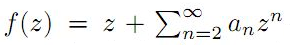, and normalized by the conditions f(0)=f'(0)-1=0. We give sharp estimates for the seventh and eighth coefficients for the class of Bazilevic functions with logarithmic growth, B1α, defined by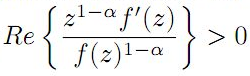for α≥0.

1: Paper Source PDF document

Paper's Title:

Solving Non-Autonomous Nonlinear Systems of Ordinary Differential Equations Using Multi-Stage Differential Transform Method

Author(s):

K. A. Ahmad, Z. Zainuddin, F. A. Abdullah

School of Mathematical Sciences Universiti Sains Malaysia
11800 USM Penang
Malaysia.
zarita@usm.my
farahaini@usm.my

Abstract:

Differential equations are basic tools to describe a wide variety of phenomena in nature such as, electrostatics, physics, chemistry, economics, etc. In this paper, a technique is developed to solve nonlinear and linear systems of ordinary differential equations based on the standard Differential Transform Method (DTM) and Multi-stage Differential Transform Method (MsDTM). Comparative numerical results that we are obtained by MsDTM and Runge-Kutta method are proposed. The numerical results showed that the MsDTM gives more accurate approximation as compared to the Runge-Kutta numerical method for the solutions of nonlinear and linear systems of ordinary differential equations

1: Paper Source PDF document

Paper's Title:

A New Relaxed b-metric Type and Fixed Point Results

Author(s):

P. Singh, V. Singh and Thokozani Cyprian Martin Jele

Department of Mathematics,
University of KwaZulu-Natal,
Private Bag X54001, Durban,
South Africa.
E-mail: singhp@ukzn.ac.za, singhv@ukzn.ac.za, thokozani.jele@nwu.ac.za

Abstract:

The purpose of this paper is to introduce a new relaxed α, β b-metric type by relaxing the triangle inequality. We investigate the effect that this generalization has on fixed point theorems.

1: Paper Source PDF document

Paper's Title:

Existence of Solution of Differential and Riemann-Liouville Equation Via Fixed Point Approach in Complex Valued b-Metric Spaces

Author(s):

K. Afassinou, A. A. Mebawondu, H. A. Abass and O. K. Narain

Department of Science Access,
South Africa.
E-mail: komia@aims.ac.za

DST-NRF Centre of Excellence in Mathematical and Statistical Sciences (CoE-MaSS),
Johannesburg,
South Africa.
E-mail: dele@aims.ac.za

DST-NRF Centre of Excellence in Mathematical and Statistical Sciences (CoE-MaSS),
Johannesburg,
South Africa.
E-mail: hammedabass548@gmail.com

School of Mathematics, Statistics and Computer Science,
University of KwaZulu-Natal, Durban,
South Africa.
E-mail: naraino@ukzn.ac.za

Abstract:

In this paper, we establish some fixed point and common fixed point results for a new type of generalized contractive mapping using the notion of C-class function in the framework of complex valued b-metric spaces. As an application, we establish the existence and uniqueness of a solution for Riemann-Liouville integral and ordinary differential equation in the framework of a complete complex valued b-metric spaces. The obtained results generalize and improve some fixed point results in the literature.

1: Paper Source PDF document

Paper's Title:

Numerical Solution of Certain Types of Fredholm-Volterra Integro-Fractional Differential Equations via Bernstein Polynomials

Author(s):

Alias B. Khalaf1, Azhaar H. Sallo2 and Shazad S. Ahmed3

1Department of Mathematics, College of Science,
University of Duhok,
Kurdistan Region,
Iraq.
E-mail:  aliasbkhalaf@uod.ac

2Department of Mathematics, College of Science,
University of Duhok,
Kurdistan Region,
Iraq.
E-mail:  azhaarsallo@uod.ac

3Department of Mathematics, College of Science,
University of Sulaimani,
Kurdistan Region,
Iraq.

Abstract:

In this article we obtain a numerical solution for a certain fractional order integro-differential equations of Fredholm-Volterra type, where the fractional derivative is defined in Caputo sense. The properties of Bernstein polynomials are applied in order to convert the fractional order integro-differential equations to the solution of algebraic equations. Some numerical examples are investigated to illustrate the method. Moreover, the results obtained by this method are compared with the exact solution and with the results of some existing methods as well.

1: Paper Source PDF document

Paper's Title:

Improved Oscillation Criteria of Second-Order Advanced Non-canonical Difference Equation

Author(s):

G. E. Chatzarakis1, N. Indrajith2, S. L. Panetsos1, E. Thandapani3

1Department of Electrical and Electronic Engineering Educators
School of Pedagogical and Technological Education,
Marousi 15122, Athens,
Greece.
E-mail: gea.xatz@aspete.gr, geaxatz@otenet.gr
spanetsos@aspete.gr

2Department of Mathematics,
Presidency College, Chennai - 600 005,
India.
E-mail: indrajithna@gmail.com

3Ramanujan Institute for Advanced Study in Mathematics,
University of Madras Chennai - 600 005,
India.
E-mail: ethandapani@yahoo.co.in

Abstract:

Employing monotonic properties of nonoscillatory solutions, we derive some new oscillation criteria for the second-order advanced non-canonical difference equation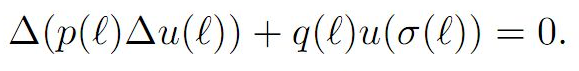Our results extend and improve the earlier ones. The outcome is illustrated via some particular difference equations.

1: Paper Source PDF document

Paper's Title:

Several New Closed-form Evaluations of the Generalized Hypergeometric Function with Argument 1/16

Author(s):

B. R. Srivatsa Kumar, Insuk Kim and Arjun K. Rathie

Department of Mathematics,
Manipal Institute of Technology,
Manipal 576 104,
India.
E-mail: sri_vatsabr@yahoo.com

Department of Mathematics Education,
Wonkwang University,
Iksan, 54538,
Republic of Korea.
E-mail: iki@wku.ac.kr

Department of Mathematics,
Vedant College of Engineering and Technology,
Rajasthan Technical University,
Bundi, 323021, Rajasthan,
India.
E-mail: arjunkumarrathie@gmail.com

Abstract:

The main objective of this paper is to establish as many as thirty new closed-form evaluations of the generalized hypergeometric function q+1Fq(z) for q= 2, 3, 4. This is achieved by means of separating the generalized hypergeometric function q+1Fq(z) for q=1, 2, 3, 4, 5 into even and odd components together with the use of several known infinite series involving central binomial coefficients obtained earlier by Ji and Hei \& Ji and Zhang.

1: Paper Source PDF document

Paper's Title:

Introducing the Picard-S3 Iteration for Fixed Points

Author(s):

Pravin Singh, Virath Singh and Shivani Singh

University of KwaZulu-Natal,
School of Mathematics Statistics and Computer Sciences,
Private Bag X54001,
Durban, 4000
South Africa.

Unisa,
Department of Decision Sciences,
PO Box 392,
Pretoria, 0003
South Africa.
E-mail: singhprook@gmail.com
singhv@ukzn.ac.za
shivstarsingh@gmail.com

Abstract:

In this paper we introduce a three step iteration method and show that it can be used to approximate the fixed point of a weak contraction mapping. Furthermore, we prove that this scheme is equivalent to the Mann iterative scheme. A comparison is made with other three step iterative methods by examining the speed of convergence. Results are presented in tables to support our conclusion.

1: Paper Source PDF document

Paper's Title:

Bounds for the Extremal Eigenvalues of Positive Definite Matrices

Author(s):

Shivani Singh and Pravin Singh

Unisa, Department of Decision Sciences,
PO Box 392,
Pretoria,
0003,
South Africa.
E-mail: singhs2@unisa.ac.za

University of KwaZulu-Natal,
School of Mathematics Statistics and Computer Sciences
Private Bag X54001,
Durban,
4000,
South Africa.
E-mail: singhprook@gmail.com

Abstract:

We use a projection to achieve bounds for a vector function of the eigenvalues of a positive definite matrix. For various choices of the monotonic function we are able to obtain bounds for the extremal eigenvalues in terms of the traces of the matrix and its powers. These bounds are relatively simple to compute.

1: Paper Source PDF document

Paper's Title:

High Order Collocation Method for the Generalized Kuramoto-Sivashinsky Equation

Author(s):

Zanele Mkhize, Nabendra Parumasur and Pravin Singh

School of Mathematics, Statistics and Computer Sciences,
University of KwaZulu-Natal,
Private Bag X 54001,
Durban 4000.
E-mail: mkhizez2@ukzn.ac.za
parumasurn1@ukzn.ac.za
singhp@ukzn.ac.za
URL: https://www.ukzn.ac.za

Abstract:

In this paper, we derive the heptic Hermite basis functions and use them as basis functions in the orthogonal collocation on finite elements (OCFE) method. We apply the method to solve the generalized Kuramoto-Sivashinsky equation. Various numerical simulations are presented to justify the computational efficiency of the proposed method.

1: Paper Source PDF document

Paper's Title:

Higher Order Accurate Compact Schemes for Time Dependent Linear and Nonlinear Convection-Diffusion Equations

Author(s):

S. Thomas, Gopika P.B. and S. K. Nadupuri

Department of Mathematics
National Institute of Technology Calicut
Kerala
673601
India.
E-mail: sobinputhiyaveettil@gmail.com pbgopika@gmail.com nsk@nitc.ac.in

Abstract:

The primary objective of this work is to study higher order compact finite difference schemes for finding the numerical solution of convection-diffusion equations which are widely used in engineering applications. The first part of this work is concerned with a higher order exponential scheme for solving unsteady one dimensional linear convection-diffusion equation. The scheme is set up with a fourth order compact exponential discretization for space and cubic \$C^1\$-spline collocation method for time. The scheme achieves fourth order accuracy in both temporal and spatial variables and is proved to be unconditionally stable. The second part explores the utility of a sixth order compact finite difference scheme in space and Huta's improved sixth order Runge-Kutta scheme in time combined to find the numerical solution of one dimensional nonlinear convection-diffusion equations. Numerical experiments are carried out with Burgers' equation to demonstrate the accuracy of the new scheme which is sixth order in both space and time. Also a sixth order in space predictor-corrector method is proposed. A comparative study is performed of the proposed schemes with existing predictor-corrector method. The investigation of computational order of convergence is presented.

1: Paper Source PDF document

Paper's Title:

Geometrical Properties of Subclass of Analytic Function with Odd Degree

Author(s):

K. Sivagami Sundari and B. Srutha Keerthi

Divison of Mathematics, School of Advanced Sciences,
Vellore Institute of Technology, Chennai Campus, Chennai - 600 127,
India.
E-mail: sivagamisundari.2298@gmail.com

Divison of Mathematics, School of Advanced Sciences,
Vellore Institute of Technology, Chennai Campus, Chennai - 600 127,
India.
E-mail: keerthivitmaths@gmail.com

Abstract:

The objective of the paper is to study the geometrical properties of the class B(λ, t). For which we have proved that the radius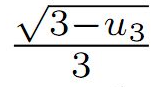is optimal ,(i.e) the numbercannot be replaced by a larger one. Additionally, the graphs for various values of t and λ are compared in order to study the sharpness of the coefficient bounds.

Search and serve lasted 0 second(s).